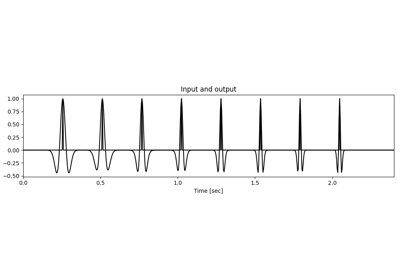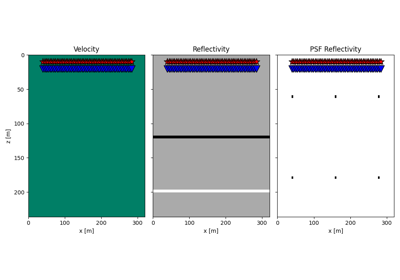# pylops.signalprocessing.NonStationaryConvolve2D#

class pylops.signalprocessing.NonStationaryConvolve2D(dims, hs, ihx, ihz, engine='numpy', num_threads_per_blocks=(32, 32), dtype='float64', name='C')[source]#

2D non-stationary convolution operator.

Apply non-stationary two-dimensional convolution. A varying compact filter is provided on a coarser grid and on-the-fly interpolation is applied in forward and adjoint modes. Both input and output have size $$n_x \times n_z$$.

Parameters
dims

Number of samples for each dimension (which we refer to as $$n_x \times n_z$$).

hsnumpy.ndarray

Bank of 2d compact filters of size $$n_{\text{filts},x} \times n_{\text{filts},z} \times n_{h,x} \times n_{h,z}$$. Filters must have odd number of samples and are assumed to be centered in the middle of the filter support.

ihxtuple

Indices of the x locations of the filters hs in the model (and data). Note that the filters must be regularly sampled, i.e. $$dh_x=\text{diff}(ihx)=\text{const.}$$

ihztuple

Indices of the z locations of the filters hs in the model (and data). Note that the filters must be regularly sampled, i.e. $$dh_z=\text{diff}(ihz)=\text{const.}$$

enginestr, optional

Engine used for spread computation (numpy, numba, or cuda)

num_threads_per_blockstuple, optional

Number of threads in each block (only when engine=cuda)

dtypestr, optional

Type of elements in input array.

namestr, optional

Name of operator (to be used by pylops.utils.describe.describe)

Raises
ValueError

If filters hs have even size

ValueError

If ihx or ihz is not regularly sampled

ValueError

If ihx or ihz are outside the bounds defined by dims

NotImplementedError

If engine is neither numpy, fftw, nor scipy.

Notes

The NonStationaryConvolve2D operator applies non-stationary two-dimensional convolution between the input signal $$d(x, z)$$ and a bank of compact filter kernels $$h(x, z; x_i, z_i)$$. Assuming an input signal composed of $$N \times M$$ samples (with $$N=4$$ and $$M=3$$, and filters at locations $$x_1, x_3$$ and $$z_1, z_3$$, the forward operator can be represented as follows:

$\begin{split}\mathbf{y} = \begin{bmatrix} \hat{h}_{(0,0),(0,0)} & \cdots & h_{(1,1),(0,0)} & \cdots & \hat{h}_{(2,2),(0,0)} & \cdots \\ \hat{h}_{(0,0),(0,1)} & \cdots & h_{(1,1),(0,1)} & \cdots & \hat{h}_{(2,2),(0,0)} & \cdots \\ \vdots & \ddots & & \ddots & \vdots & \vdots \\ \hat{h}_{(0,0),(4,3)} & \cdots & h_{(1,1),(4,3)} & \cdots & \hat{h}_{(2,2),(0,0)} & \cdots \\ \end{bmatrix} \begin{bmatrix} x_{0,0} \\ \vdots \\ x_{0,N} \\ x_{1,0} \\ \vdots \\ x_{1,N} \\ x_{M,0} \\ \vdots \\ x_{M,N} \end{bmatrix}\end{split}$

where $$\mathbf{h}_{(1,1)} = [h_{(1,1),(0,0)}, h_{(1,1),(0,1)}, \ldots, h_{(1,1),(4,3)}]$$ (and $$\mathbf{h}_{(1,1)}$$, $$\mathbf{h}_{(1,3)}$$, $$\mathbf{h}_{(3,1)}$$, $$\mathbf{h}_{(3,3)}$$) are the provided filter, $$\hat{\mathbf{h}}_{(0,0)} = \mathbf{h}_{(1,1)}$$ and similar are the filters outside the range of the provided filters (which are extrapolated to be the same as the nearest provided filter) and $$\hat{\mathbf{h}}_{(2,2)} = \text{bilinear}(\mathbf{h}_{(1,1)}, \mathbf{h}_{(3,1)}, \mathbf{h}_{(1,3)},\mathbf{h}_{(3,3)})$$ is the filter within the range of the provided filters (which is bilinearly interpolated from the four nearest provided filter on either side of its location).

For more details on the numerical implementation of the forward and adjoint, see pylops.signalprocessing.NonStationaryConvolve1D.

Attributes
shapetuple

Operator shape

explicitbool

Operator contains a matrix that can be solved explicitly (True) or not (False)

Methods

 __init__(dims, hs, ihx, ihz[, engine, ...]) adjoint() apply_columns(cols) Apply subset of columns of operator cond([uselobpcg]) Condition number of linear operator. conj() Complex conjugate operator div(y[, niter, densesolver]) Solve the linear problem $$\mathbf{y}=\mathbf{A}\mathbf{x}$$. dot(x) Matrix-matrix or matrix-vector multiplication. eigs([neigs, symmetric, niter, uselobpcg]) Most significant eigenvalues of linear operator. matmat(X) Matrix-matrix multiplication. matvec(x) Matrix-vector multiplication. reset_count() Reset counters rmatmat(X) Matrix-matrix multiplication. rmatvec(x) Adjoint matrix-vector multiplication. todense([backend]) Return dense matrix. toimag([forw, adj]) Imag operator toreal([forw, adj]) Real operator tosparse() Return sparse matrix. trace([neval, method, backend]) Trace of linear operator. transpose()

## Examples using pylops.signalprocessing.NonStationaryConvolve2D#Non-stationary Convolution

Non-stationary Convolution20. Image Domain Least-squares migration

20. Image Domain Least-squares migration